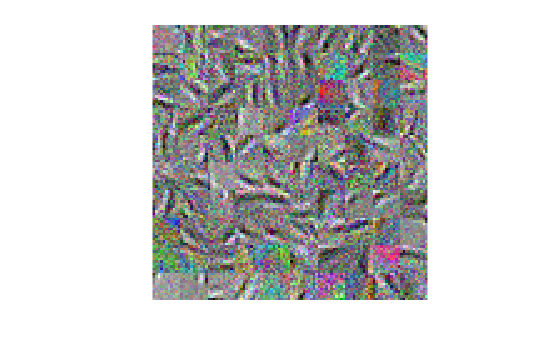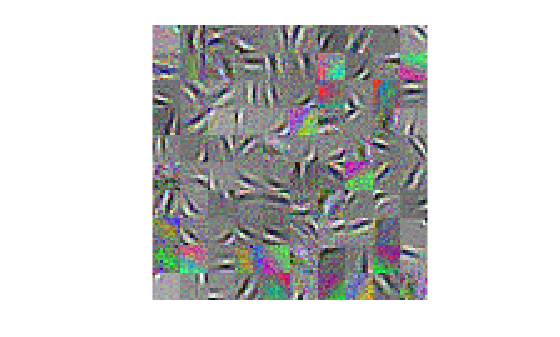# sparsefilt

Feature extraction by using sparse filtering

## Syntax

``Mdl = sparsefilt(X,q)``
``Mdl = sparsefilt(X,q,Name,Value)``

## Description

````Mdl = sparsefilt(X,q)` returns a sparse filtering model object that contains the results from applying sparse filtering to the table or matrix of predictor data `X` containing p variables. `q` is the number of features to extract from `X`, therefore `sparsefilt` learns a p-by-`q` matrix of transformation weights. For undercomplete or overcomplete feature representations, `q` can be less than or greater than the number of predictor variables, respectively. To access the learned transformation weights, use `Mdl.TransformWeights`.To transform `X` to the new set of features by using the learned transformation, pass `Mdl` and `X` to `transform`. ```

example

````Mdl = sparsefilt(X,q,Name,Value)` uses additional options specified by one or more `Name,Value` pair arguments. For example, you can standardize the predictor data or apply L2 regularization.```

## Examples

collapse all

Create a `SparseFiltering` object by using the `sparsefilt` function.

Load the `SampleImagePatches` image patches.

```data = load('SampleImagePatches'); size(data.X)```
```ans = 1×2 5000 363 ```

There are 5,000 image patches, each containing 363 features.

Extract 100 features from the data.

```rng default % For reproducibility Q = 100; obj = sparsefilt(data.X,Q,'IterationLimit',100)```
```Warning: Solver LBFGS was not able to converge to a solution. ```
```obj = SparseFiltering ModelParameters: [1x1 struct] NumPredictors: 363 NumLearnedFeatures: 100 Mu: [] Sigma: [] FitInfo: [1x1 struct] TransformWeights: [363x100 double] InitialTransformWeights: [] Properties, Methods ```

`sparsefilt` issues a warning because it stopped due to reaching the iteration limit, instead of reaching a step-size limit or a gradient-size limit. You can still use the learned features in the returned object by calling the `transform` function.

Continue optimizing a sparse filter.

Load the `SampleImagePatches` image patches.

```data = load('SampleImagePatches'); size(data.X)```
```ans = 1×2 5000 363 ```

There are 5,000 image patches, each containing 363 features.

Extract 100 features from the data and use an iteration limit of 20.

```rng default % For reproducibility q = 100; Mdl = sparsefilt(data.X,q,'IterationLimit',20);```
```Warning: Solver LBFGS was not able to converge to a solution. ```

View the resulting transformation matrix as image patches.

```wts = Mdl.TransformWeights; W = reshape(wts,[11,11,3,q]); [dx,dy,~,~] = size(W); for f = 1:q Wvec = W(:,:,:,f); Wvec = Wvec(:); Wvec =(Wvec - min(Wvec))/(max(Wvec) - min(Wvec)); W(:,:,:,f) = reshape(Wvec,dx,dy,3); end m = ceil(sqrt(q)); n = m; img = zeros(m*dx,n*dy,3); f = 1; for i = 1:m for j = 1:n if (f <= q) img((i-1)*dx+1:i*dx,(j-1)*dy+1:j*dy,:) = W(:,:,:,f); f = f+1; end end end imshow(img,'InitialMagnification',300);```The image patches appear noisy. To clean up the noise, try more iterations. Restart the optimization from where it stopped for another 40 iterations.

`Mdl = sparsefilt(data.X,q,'IterationLimit',40,'InitialTransformWeights',wts);`
```Warning: Solver LBFGS was not able to converge to a solution. ```

View the updated transformation matrix as image patches.

```wts = Mdl.TransformWeights; W = reshape(wts,[11,11,3,q]); [dx,dy,~,~] = size(W); for f = 1:q Wvec = W(:,:,:,f); Wvec = Wvec(:); Wvec =(Wvec - min(Wvec))/(max(Wvec) - min(Wvec)); W(:,:,:,f) = reshape(Wvec,dx,dy,3); end m = ceil(sqrt(q)); n = m; img = zeros(m*dx,n*dy,3); f = 1; for i = 1:m for j = 1:n if (f <= q) img((i-1)*dx+1:i*dx,(j-1)*dy+1:j*dy,:) = W(:,:,:,f); f = f+1; end end end imshow(img,'InitialMagnification',300);```These images are less noisy.

## Input Arguments

collapse all

Predictor data, specified as an n-by-p numeric matrix or table. Rows correspond to individual observations and columns correspond to individual predictor variables. If `X` is a table, then all of its variables must be numeric vectors.

Data Types: `single` | `double` | `table`

Number of features to extract from the predictor data, specified as a positive integer.

`sparsefilt` stores a p-by-`q` transform weight matrix in `Mdl.TransformWeights`. Therefore, setting very large values for `q` can result in greater memory consumption and increased computation time.

Data Types: `single` | `double`

### Name-Value Arguments

Specify optional pairs of arguments as `Name1=Value1,...,NameN=ValueN`, where `Name` is the argument name and `Value` is the corresponding value. Name-value arguments must appear after other arguments, but the order of the pairs does not matter.

Before R2021a, use commas to separate each name and value, and enclose `Name` in quotes.

Example: `'Standardize',true,'Lambda',1` standardizes the predictor data and applies a penalty of `1` to the transform weight matrix.

Maximum number of iterations, specified as the comma-separated pair consisting of `'IterationLimit'` and a positive integer.

Example: `'IterationLimit',1e6`

Data Types: `single` | `double`

Verbosity level for monitoring algorithm convergence, specified as the comma-separated pair consisting of `'VerbosityLevel'` and a value in this table.

ValueDescription
`0``sparsefilt` does not display convergence information at the command line.
Positive integer`sparsefilt` displays convergence information at the command line.

Convergence Information

`FUN VALUE`Objective function value.
`NORM GRAD`Norm of the gradient of the objective function.
`NORM STEP`Norm of the iterative step, meaning the distance between the previous point and the current point.
`CURV``OK` means the weak Wolfe condition is satisfied. This condition is a combination of sufficient decrease of the objective function and a curvature condition.
`GAMMA`Inner product of the step times the gradient difference, divided by the inner product of the gradient difference with itself. The gradient difference is the gradient at the current point minus the gradient at the previous point. Gives diagnostic information on the objective function curvature.
`ALPHA`Step direction multiplier, which differs from `1` when the algorithm performed a line search.
`ACCEPT``YES` means the algorithm found an acceptable step to take.

Example: `'VerbosityLevel',1`

Data Types: `single` | `double`

L2 regularization coefficient value for the transform weight matrix, specified as the comma-separated pair consisting of `'Lambda'` and a positive numeric scalar. If you specify `0`, the default, then there is no regularization term in the objective function.

Example: `'Lambda',0.1`

Data Types: `single` | `double`

Flag to standardize the predictor data, specified as the comma-separated pair consisting of `'Standardize'` and `true` (`1`) or `false` (`0`).

If `Standardize` is `true`, then:

• `sparsefilt` centers and scales each column of the predictor data (`X`) by the column mean and standard deviation, respectively.

• `sparsefilt` extracts new features by using the standardized predictor matrix, and stores the predictor variable means and standard deviations in properties `Mu` and `Sigma` of `Mdl`.

Example: `'Standardize',true`

Data Types: `logical`

Transformation weights that initialize optimization, specified as the comma-separated pair consisting of `'InitialTransformWeights'` and a p-by-`q` numeric matrix. p must be the number of columns or variables in `X` and `q` is the value of `q`.

Tip

You can continue optimizing a previously returned transform weight matrix by passing it as an initial value in another call to `sparsefilt`. The output model object `Mdl` stores a learned transform weight matrix in the `TransformWeights` property.

Example: `'InitialTransformWeights',Mdl.TransformWeights`

Data Types: `single` | `double`

Relative convergence tolerance on gradient norm, specified as the comma-separated pair consisting of `'GradientTolerance'` and a positive numeric scalar. This gradient is the gradient of the objective function.

Example: `'GradientTolerance',1e-4`

Data Types: `single` | `double`

Absolute convergence tolerance on the step size, specified as the comma-separated pair consisting of `'StepTolerance'` and a positive numeric scalar.

Example: `'StepTolerance',1e-4`

Data Types: `single` | `double`

## Output Arguments

collapse all

Learned sparse filtering model, returned as a `SparseFiltering` model object.

To access properties of `Mdl`, use dot notation. For example:

• To access the learned transform weights, use `Mdl.TransformWeights`.

• To access the fitting information structure, use `Mdl.FitInfo`.

To find sparse filtering coefficients for new data, use the `transform` function.

## Algorithms

The `sparsefilt` function creates a nonlinear transformation of input features to output features. The transformation is based on optimizing an objective function that encourages the representation of each example by as few output features as possible while at the same time keeping the output features equally active across examples.

For details, see Sparse Filtering Algorithm.

## Version History

Introduced in R2017a